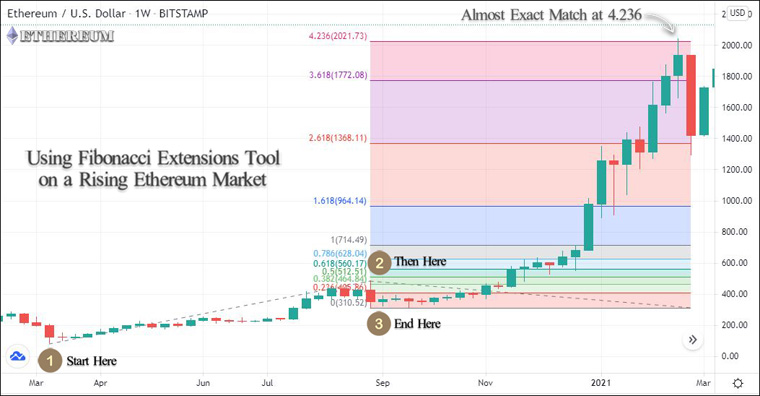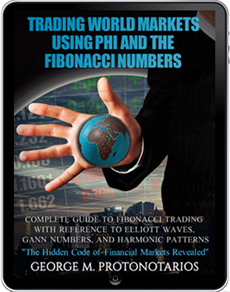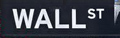Introduction to Fibonacci Mathematics

Fibonacci mathematics can help traders to reveal the hidden proportionality of market behavior. Fibonacci extension analysis studies the extends of prime trends and countertrends in order to identify key reversal zones, or else levels where a trending market may lose momentum and reverse.

Calculating the Basic Ratios using the Fibonacci Sequence

The Fibonacci sequence of numbers begins as follows: 0, 1, 2, 3, 5, 8, 13, 21, 34, 55, 89, 144, 233 and etc.

The above sequence can then be broken down into ratios. The Fibo ratios can be found by dividing the Fibonacci numbers into each other.

If we do the math by excluding the first numbers, we realize that:

• Every number is approximately 1.618 times the preceding number
• Every number is 0.618 of the number to the right of it

Note that 1.618 is the golden ratio, and its inverse is 0.618.

In Financial trading the key ratios are 0.236, 0.382, 0.618, 1.618, 2.618, and 4.236. Many traders also use 0.5, 1.0.

Table: Key Fibonacci ratios for Financial trading

 0.236 1 0.382 1.618 0.5 2.618 0.618 3.618 0.786 4.236

Fibonacci Extensions

The Fibonacci sequence of numbers produces useful trading tools such as the Fibonacci retracement and the Fibonacci extensions.

Fibonacci extensions can identify the extends of prime trends and countertrends in order to spot potential price reversal zones. Let's start by calculating the Fibo extensions above 100.

Calculating Fibonacci Extensions above 100%

These are some basic calculations, as presented in the following table:

• A, the Fibonacci sequence
• A1, dividing each Fibonacci number by the prior number (1 position), and the ratio approaches 1.618
• A2, dividing each Fibonacci number by two places to the left (2 positions), and the ratio approaches 2.618
• A3, dividing each Fibonacci number by three places to the left (3 positions), and the ratio approaches 4.236

Table: Calculating all Fibonacci Ratios

 A A1 A2 A3 1 2 2.000 (2/1) 3 1.500 (3/2) 3.000 (3/1) 5 1.667 (5/3) 2.500 (5/2) 5.000 (5/1) 8 1.600 (8/5) 2.667 (8/3) 4.000 (8/2) 13 1.625 (13/8) 2.600 (13/5) 4.333 (13/3) 21 1.615 2.625 4.200 34 1.619 2.615 4.250 55 1.618 2.619 4.231 89 1.618 2.618 4.238 144 1.618 2.618 4.235 233 1.618 2.618 4.236 377 1.618 2.618 4.236

By adding 3.618 (2.618+1), the key Fibonacci extensions (above 100) are 161.8%, 261.8%, 361.8%, and 423.6%.

Usage

Extension levels are areas where the price is expected to reverse. The Trend Based Fibonacci Extensions (TBFEs) are drawn on any chart and work through the use of the Fibonacci ratios. In bull markets, the Fibonacci Extensions tool is particularly useful in order to determine strong resistance when the price of an asset is found at price discovery. However, you can apply the tool on a bearish market as well. The Fibonacci Extensions tool can be used for multiple purposes:

• Evaluating how far primary uptrends and downtrends can go.
• Creating useful targets for our orders (take-profit and stop-loss), especially when the price of an asset is found at price discovery.
• Analyzing price corrections and distinguishing between temporary price pullbacks and key trend reversals.
• Analyzing crowd behavior during extremely bullish market movements, when other TA tools fail.

How to Draw the Fibonacci Levels

Fibonacci Extensions are drawn by joining three (3) points, in contrast to the Fibonacci Retracement which has only (2) two points. The first thing is to spot a trend that will be used as a base.

In order to draw the Trend Based Fibonacci Extensions in a bullish trend, you need to click on three separate price levels: the start and the end of the prime trend, plus the end of the secondary end. In a bearish trend, the logic remains the same, but in reverse.

• Click-1: Start by clicking on the beginning of the price movement
• Click-2: Click on the point of completion of the price movement
• Click-2: Click on the point of completion of the secondary trend (end of the retracement against that move)

In the following chart, applying the Trend-Based Fibonacci Extension (TBFE) on Ethereum/USD.

Image: Trend-Based Fibonacci Extension Tool on Ethereum (TradingView)Fibonacci Extensions use the same logic as the Fibonacci Retracement. Both tools indicate levels of a potential trend reversal. The main difference is that Fibonacci extensions can analyze trends that extend beyond the base trend, and that means the price extends above the 100% level. That is a common situation for recently-listed financial assets lacking historical trading data or for financial assets that move in price discovery after a significant fundamental shift.

Fibonacci extensions can signal entries when the price bounces from an extension level, or indicate take-profit price levels:

• Use the Fibonacci extension levels like any other support and resistance levels.
• Trade in the direction of the trend when there is a breakout of a Fibonacci extension level. Take profits near the next extension level.
• Trade a trend reversal, after price bounces from an extension level.
• Create very useful take-profit zones, close to Fibonacci extensions levels. In addition, indicate price targets when the price of an asset is at price discovery.
• Enter a Stop-Loss order near the next Fibonacci extension level.

Key Takeaways

• Fibonacci Extensions apply the same logic as the Fibonacci Retracement, however, Fibonacci extensions can analyze trends that extend beyond the base trend, and that means the price extends above the 100% level.
• The key extensions above 100 are 161.8%, 261.8%, 361.8%, and 423.6%.
• In bullish trends, the Fibonacci Extensions tool is particularly useful in order to determine strong resistance when the price of an asset is found at price discovery.
• The Trend Based Fibonacci Extensions (TBFEs) are drawn on any chart and work through the use of the Fibonacci ratios.
• In order to draw the Fib Extensions, you need a trend and two swing points.
• Extension levels are price zones where the trend is likely to reverse.
• You can use the Fibonacci extensions as price targets. Profit-taking can include various sell orders spread across different Fibonacci extensions.
• You can use multiple orders based on Fibonacci Extension levels, but you should remember that these levels indicate a zone of support/resistance, not exact points.
• False signals can always appear.
• Prefer to apply the Fibonacci Extensions tool in higher timeframes, and wait for an official closing price.
• Traders should use Fibonacci Extensions in combination with another indicator or a continuation/reversal pattern in a higher timeframe.

Introduction to Fibonacci Extensions### Online Forex BrokersFibonacci mathematics aims to reveal the hidden proportionality of market behavior. Find Fibonacci trading tools and tutorials:

eBOOKTRADING WORLD MARKETS USING PHI AND THE FIBONACCI NUMBERS (2018)

Complete Guide to Fibonacci Trading with Reference to Elliott Waves, Gann Numbers, and Harmonic Patterns### 2022 HolidaysDate Holiday Jan. 17, Monday Martin Luther King Feb. 21, Monday Presidents' Day April 15, Friday Good Friday May 30, Monday Memorial Day June 20, Monday Juneteenth Day July 4, Monday Independence Day Sept. 5, Monday Labor Day Nov. 24, Thursday Thanksgiving Day Dec. 26, Monday Christmas Day

### Chart PatternsIdentify and Trade Reliable Chart Patterns: